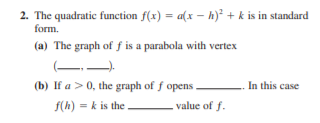# 2. The quadratic function f(x) = a(x – h)² + k is in standard form. (a) The graph of ƒ is a parabola with vertex (b) If a >0, the graph of f opens In this case f(h) = k is the value of f.

Question

The quadratic function f1x2  a1x  h2
2  k is in standard
form.
(a) The graph of f is a parabola with vertex
1 , 2.
(b) If a  0, the graph of f opens . In this case
f1h2  k is the value of f.help_outlineImage Transcriptionclose2. The quadratic function f(x) = a(x – h)² + k is in standard form. (a) The graph of ƒ is a parabola with vertex (b) If a >0, the graph of f opens In this case f(h) = k is the value of f. fullscreen

### Want to see this answer and more?

Experts are waiting 24/7 to provide step-by-step solutions in as fast as 30 minutes!*

*Response times may vary by subject and question complexity. Median response time is 34 minutes for paid subscribers and may be longer for promotional offers.
Tagged in
Math
Algebra

### Other